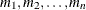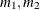### PARMS Statement

`PARMS` <(value-list)…> </ options> ;

The PARMS statement specifies initial values for the covariance parameters, or it requests a grid search over several values of these parameters. You must specify the values in the order in which they appear in the Covariance Parameter Estimates table.

The value-list specification can take any of several forms:

m

a single valueseveral values

m to n

a sequence where m equals the starting value, n equals the ending value, and the increment equals 1

m to n by i

a sequence where m equals the starting value, n equals the ending value, and the increment equals itomixed values and sequences

You can use the PARMS statement to input known parameters. Suppose the three variance components are known to be 2, 1, and 3. The SAS statements to fix the variance components at these values are as follows:

```proc hpmixed noprofile;
class Family Gender;
model Height = Gender;
random Family Family*Gender;
parms (2) (1) (3) / noiter;
run;
```

The NOPROFILE option in the PROC HPMIXED statement suppresses profiling the residual variance parameter during its calculations, thereby enabling its value to be held at 3 as specified in the PARMS statement.

If you specify more than one set of initial values, PROC HPMIXED performs a grid search of the likelihood surface and uses the best point on the grid for subsequent analysis. Specifying a large number of grid points can result in long computing times. The grid search feature is also useful for exploring the likelihood surface.

The results from the PARMS statement are the values of the parameters on the specified grid (denoted by CovP1–CovPn), the residual variance (possibly estimated) for models with a residual variance parameter, and various functions of the likelihood.

The name of the Parameter Search table is ParmSearch.

You can specify the following options in the PARMS statement after a slash (/).

HOLD=value-list
HOLD

specifies which parameter values PROC HPMIXED should hold to equal the specified values. To hold all parameters, you can use the second form without giving the value-list. For example, the following statement constrains the first and third covariance parameters to equal 5 and 2, respectively.

Specifying the HOLD= option implies the NOPROFILE option in the PROC HPMIXED statement:

```parms (5) (3) (2) (3) / hold=1,3;
```
LOWERB=value-list

enables you to specify lower boundary constraints on the covariance parameters. The value-list specification is a list of numbers or missing values (.) separated by commas. You must list the numbers in the order that PROC HPMIXED uses for the covariance parameters, and each number corresponds to the lower boundary constraint. A missing value instructs PROC HPMIXED to use its default constraint, and if you do not specify numbers for all of the covariance parameters, PROC MIXED assumes the remaining ones are missing.

NOITER

requests that no optimization iterations be performed and that PROC HPMIXED use the best value from the grid search to perform inferences. By default, iterations begin at the best value from the PARMS grid search. This option is ignored when you specify the HOLD= option.

If a residual variance is profiled, the parameter estimates can change from the initial values you provide as the residual variance is recomputed. To prevent an update of the residual variance, combine the NOITER option with the NOPROFILE option in the PROC HPMIXED statements, as in the following program:

```proc hpmixed noprofile;
class A B C rep mp sp;
model y = A | B | C;
random rep mp sp;
parms (180) (200) (170) (1000) / noiter;
run;
```

Specifying the NOITER option in the PARMS statement has the same effect as specifying TECHNIQUE=NONE in the NLOPTIONS statement.

Notice that the NOITER option can be useful if you want to obtain the starting values HPMIXED computes. The following statements produce the starting values:

```proc hpmixed noprofile;
class A B;
model y = A;
random int / subject=B;
parms / noiter;
run;
```
PARMSDATA=SAS-data-set
PDATA=SAS data set

reads in covariance parameter values from a SAS data set. The data set should contain the numerical variable ESTIMATE or the numerical variables `Covp`1–`Covp`q, where q denotes the number of covariance parameters.

If the PARMSDATA= data set contains multiple sets of covariance parameters, the HPMIXED procedure evaluates the initial objective function for each set and commences the optimization step by using the set with the lowest function value as the starting values. For example, the following SAS statements request that the objective function be evaluated for three sets of initial values:

```data data_covp;
input covp1-covp4;
datalines;
180 200 170 1000
170 190 160  900
160 180 150  800
;
proc hpmixed;
class A B C rep;
model yield = A;
random rep B C;
parms / pdata=data_covp;
run;
```

Each set comprises four covariance parameters.

The order of the observations in a data set with the numerical variable `Estimate` corresponds to the order of the covariance parameters in the Covariance Parameter Estimates table.

The PARMSDATA= data set must contain at least one set of covariance parameters with no missing values.

If the HPMIXED procedure is processing the input data set in BY groups, you can add the BY variables to the PARMSDATA= data set. If this data set is sorted by the BY variables, the HPMIXED procedure matches the covariance parameter values to the current BY group. If the PARMSDATA= data set does not contain all BY variables, the data set is processed in its entirety for every BY group and a message is written to the log. This enables you to provide a single set of starting values across BY groups, as in the following statements:

```data data_covp;
input covp1-covp4;
datalines;
180 200 170 1000
;
proc hpmixed;
class A B C rep;
model yield = A;
random rep B C;
parms / pdata=data_covp;
by year;
run;
```

The same set of starting values is used for each value of the `year` variable.

UPPERB=value-list

enables you to specify upper boundary constraints on the covariance parameters. The value-list specification is a list of numbers or missing values (.) separated by commas. You must list the numbers in the order that PROC HPMIXED uses for the covariance parameters, and each number corresponds to the upper boundary constraint. A missing value instructs PROC HPMIXED to use its default constraint, and if you do not specify numbers for all of the covariance parameters, PROC HPMIXED assumes that the remaining ones are missing.